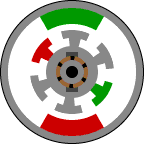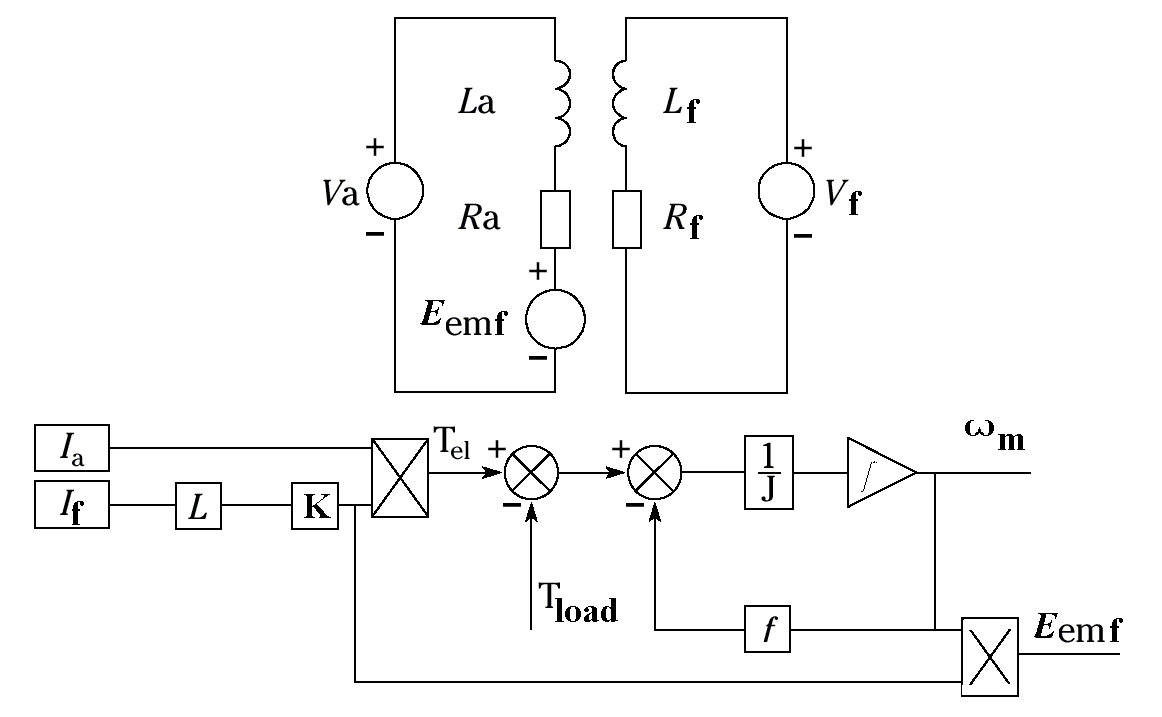• Introduction
• What is in this manual
• What is Caspoc
• User interface
• Introduction
• Starting
• Simulation
• Editing
• Viewing and printing
• Getting Started
• Basic editing
• Simulation in the time domain
• Basic User Interface Topics
• Editing
• Simulation
• Viewing
• Library
• Reports
• Project management
• Circuit and Block Diagram Components
• Introduction
• Cscript and user defined functions
• Component parameters
• Modeling Topics
• Introduction
• Power Electronics
• Semiconductors
• Electrical Machines
• Electrical drives
• Power Systems
• Mechanical Systems
• Thermal Systems
• Magnetic Circuits
• Green Energy
• Coupling to FEM
• Experimenter
• Analog hardware description language
• Embedded C code Export
• Coupling to Spice
• Small Signal Analysis
• Matlab coupling
• Tips and tricks
• Appendices

## DC MotorsClick to Close this View Many people are familiar with DC motors. If you have ever had a electric toy train or car as a child, you may know how a DC motor works. If you were like me you probably took one apart and couldn't put it back together. Usually the brush springs got lost. The stator is the stationary outside part of a motor. The rotor is the inner part which rotates. In the motor animations, red represents a magnet or winding with a north polarization, while green represents a magnet or winding with a south polarization. Opposite, red and green, polarities attract.

The stator of a permanent magnet dc motor is composed of two or more permanent magnet pole pieces. The rotor is composed of windings which are connected to a mechanical commutator. In this case the rotor has three pole pairs. The opposite polarities of the energized winding and the stator magnet attract and the rotor will rotate until it is aligned with the stator. Just as the rotor reaches alignment, the brushes move across the commutator contacts and energize the next winding. In the animation the commutator contacts are brown and the brushes are dark gray. A yellow spark shows when the brushes switch to the next winding.

Notice that the commutator is staggered from the rotor poles. If the connections of a dc motor are reversed the motor will change directions. Though it will not always work as well in both directions.

This is a permanent magnet dc motor. Two other types of dc motors are series wound and shunt wound dc motors. These motors also use a similar rotor with brushes and a commutator. However, the stator uses windings instead of permanent magnets. The basic principle is still the same. A series wound dc motor has the stator windings in series with the rotor. A shunt wound dc motor has the stator windings in parallel with the rotor winding. The series wound motor is more common. A series wound motor is also called a universal  motor. It is universal in the sense that it will run equally well using either an ac or a dc voltage source. Reversing the polarity of both the stator and the rotor cancel out. Thus the motor will always rotate the same direction irregardless of the voltage polarity. A universal motor is in a sense an ac motor in that it will operate from an ac power source. I prefer the term universal motor to avoid confusion with ac induction motors.

The DCM block in Caspoc implements a separately excited DC machine. This block contains the basic model as explained below. This block has block-diagram connections and has to be connected to an electric circuit by using two VOLTAGE blocks.

The DC machine library blocks, have electrical and mechanical terminals. There are models for DC separately wound, shunt, series, universal and permanent magnet. The model of the universal motor is in general equal to the series motor.

The equivalent circuit for modeling the DC machine is shown below:The armature circuit consists of an inductor La and resistor Ra in series with a electromotive force EEMF. The electromotive force EEMF is proportional to the machine speed ωm:

EEMF=KE · ωm

where KE is the voltage constant in [V/(rad/s)] and ωm is the machine speed in [rad/s]. In a separately excited DC machine model, the stator field Φ is proportional to the field current If:

Φ=Lf · if

where Lf is the field inductance.

The electromechanical torque developed by the DC machine is proportional to the armature current Ia and stator field:

Tel=KT · Lf · if

where KT is the torque constant. The torque constant KT is equal to the voltage constant KE and is denoted by the parameter K.

Mechanical part:

The library blocks for the DC machines have electrical and mechanical connections. The electrical terminals are dependent on the type of the DC machine, being a shunt, series or separately excited machine.
The mechanical shaft is modeled by a rigid shaft model. Only the inertia J [kgm2]of the shaft can be modeled in the DC basic blocks. Most machine models have internal parameters for modeling the inertia and friction of the shaft. The DC machine can either run without load or can be connected to a mechanical rotational model. Use the models from components/circuit/rotational and from the various mechanical libraries for modeling the mechanical model.

The parameters for the DC Machine can be modified in each library model of the DC machine, being the shunt, series, universal, or permanent magnet motor.

 Parameter SI unit Armature resistance Ra Ω Armature inductance La Henry Field resistance Ra Ω Field inductance La Henry Rotor inertia kg.m2
The friction of the rotor is defined by the mechaincal model connected to the shaft of the DC machine.